## Learning Machine Learning | Week 1 | Machine Learning Course | Andrew Ng | Coursera

Hi, so if you have read this blog, then you probably know that I had recently applied and got a financial aid from Coursera on Andrew Ng's Machine Learning Course .

I have just completed it's first week and in this blog I will share what I have learnt throughout the period so that if someday you decide to get into the course then you don't go blindfolded .

So, before I start going through it I would like to say that this course is literally Machine Learning from scratch because here you will  learn the math behind the fancy predictions and thus you will know what actually happens under the hood .And Andrew makes it very easy to understand with his calm and soothing explanations .

So, don't blindly believe, if someone says it is highly mathematically complex.

The INTRO Section :

So, in the first section you will be welcomed warmly into the world of machine learning and you  will learn the introductory materials related to ML like :

What is MACHINE LEARNING  ? :

As I have mentioned the question let me give you two answers which I found very beautiful :

" It is the field of study that gives computer the ability to learn without being explicitly programmed ." - Arthur Samuel

Now time for a technical definition :

" A computer program is said to learn from experience E with respect to some class of tasks T and the performance measure P ,if it's performance at tasks in T, as measured by P, improves with experience E. " - Tom Mitchell

What is SUPERVISED and UNSUPERVISED Learning ?

Supervised Learning :
In Supervised Learning, the data is well labeled and we know that - for this input that is the output or we have well defined input-output pairs from which the machine could understand the relations and thus will be able to predict when provided a completely new input.

Problems based on Supervised Learning are classified into two types :

Regression :

In regression we predict results within a continuous output or function . For example - Predicting cost of buying a house based on the it's area . It's output is continuous as it is dependent on some variable like in this case, area and the output i.e price continuously varies according to it .

Classification :
Here we predict discrete output or literally classify things like for example - given a picture identify whether it is a dog or cat .

Simple right !Regression (Left) & Clustering (Right)

Unsupervised Learning :

Her we don't a have a well defined input output pair, so we can't draw any conclusion directly from the data but as we train it we look for structures and patterns in the data and try to cluster them , this is called Clustering which is a type of Unsupervised learning .

For example - Analyzing frequencies or radio signals from outer space to find out patterns and cluster similar segments .

Next you start learning about Models and the math formulae behind prediction .

Model Representation :

This is how a model is represented :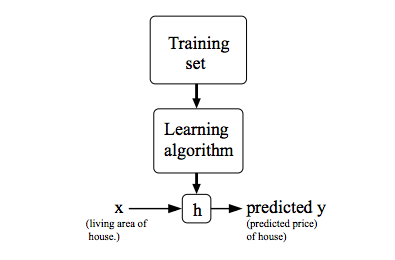where :

x= the input training data

y= the output of the respective x

h= this is called the Hypothesis Function

The Hypothesis Function, h :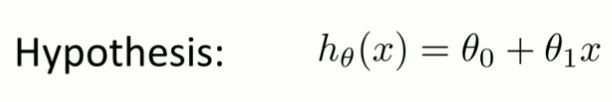And , to measure the accuracy of this hypothesis we need the Cost Function :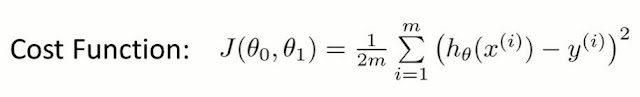This Cost function is also called the Squared error or the Mean Squared Error function .

Because if you look at the function closely, the square of  all the m hypothesis functions are summed up and  divided by m  that is how we get the mean of the squares and later we half it by multiplying 1/2 for an efficient Gradient descent about which you will learn in the next section .

Next Andrew Sir, will give two lectures where he will go deep into the cost function where he will explain all the questions that you might have related to this function like why and how it works and thus will try to build an intuition in you .

A small review :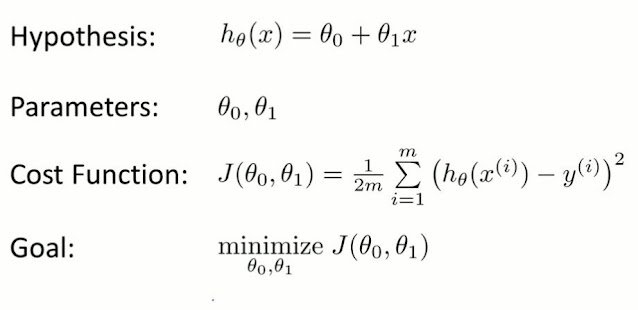We minimize the cost function using Gradient descent algorithm.

Okay now we have to estimate the parameters in the hypothesis function , this is where gradient descent algorithm comes in and this is how it goes :

θj:=θjαθjJ(θ0,θ1)

where ,
θ = jth parameter

α= Learning Rate

If you observe the part next to the 'α' it is just the derivative of the cost function or you can also say it's the slope .

So, simply put what this algorithm does is it keeps changing the value of parameters according to the learning rate and thus tries to reach a global minimum value and thus the learning rate plays an important role in this process .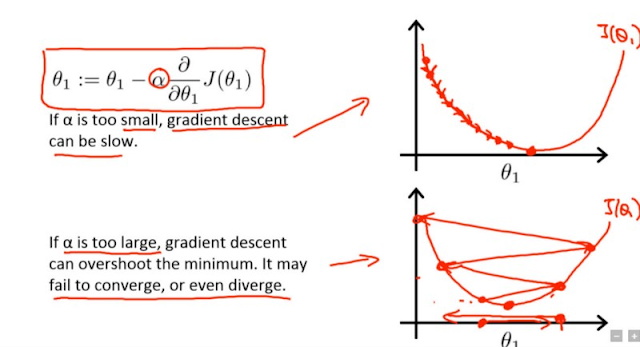Now that we have a good idea of Gradient Descent lets learn how to apply Gradient descent to Linear Regression .

Applying Gradient Descent To Linear Regression :

This just means that we substitute the linear regression cost function in the gradient descent equation  thus this is what we get as a result :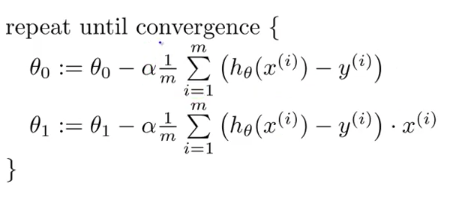The part next to the learning rate is nothing but what we get when we differentiate the cost function with respect to theta. Common let's do some math :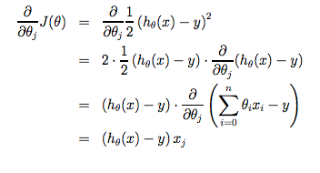At the end of the Week 1, you will appear a quiz on all the things you learnt till now and ya, at the end you have an extra section where you can brush up your Linear Algebra skills .

That is all what you learn in Week 1 of Andrew Ng's Machine Learning Stanford Course .
I hope you got a good idea of the concepts and how the first week is going to be .

Thanks for making it so far .You are awesome !!